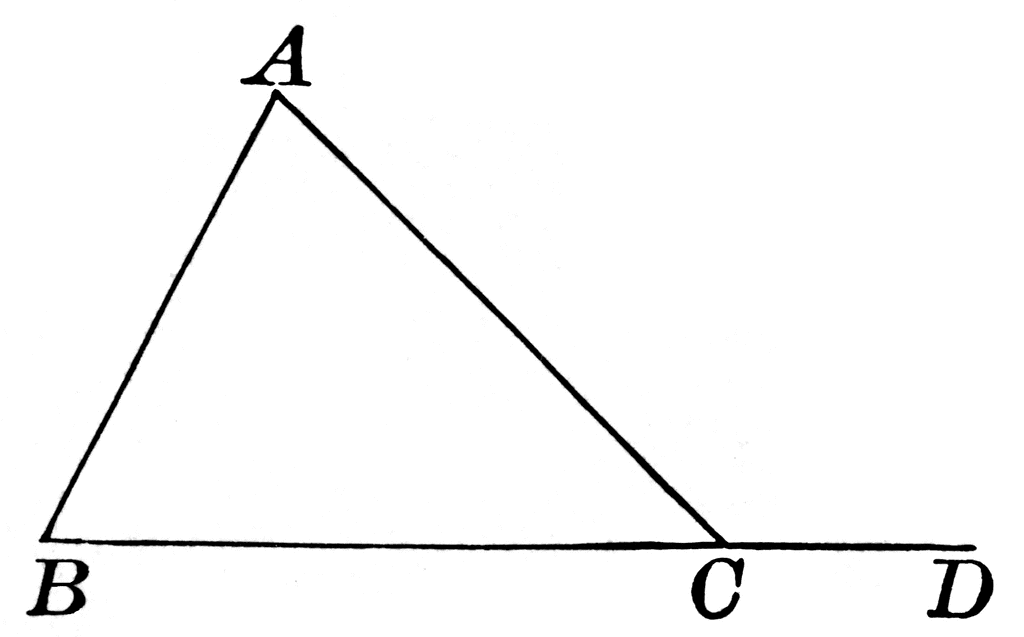Parallel linesParallel lines

In geometryparallel lines are lines in a plane which do not meet; that is, two lines in a plane that do not intersect or touch each other at any point are said to be parallel. By extension, a line and a plane, or two planes, in three-dimensional Euclidean space that do not share a point are said to be parallel. However, two lines in three-dimensional space which do not meet must be in a common plane to be considered parallel; otherwise they are called skew lines. Parallel planes are planes in the same three-dimensional space that never meet.

Parallel lines are the subject of Euclid‘s parallel postulate. Parallelism is primarily a property of affine geometries and Euclidean geometry is a special instance of this type of geometry. In some other geometries, such as hyperbolic geometry, lines can have analogous properties that are referred to as parallelism.

Interior anglesIn geometry, an angle of a polygon is formed by two sides of the polygon that share an endpoint. For a simple (non-self-intersecting) polygon, regardless of whether it is convex or non-convex, this angle is called an interior angle (or internal angle) if a point within the angle is in the interior of the polygon. A polygon has exactly one internal angle per vertex.

If every internal angle of a simple polygon is less than 180°, the polygon is called convex.

In contrast

Exterior anglesan exterior angle (or external angle) is an angle formed by one side of a simple polygon and a line extended from an adjacent side.:pp. 261-264